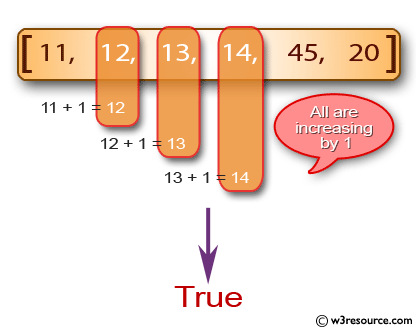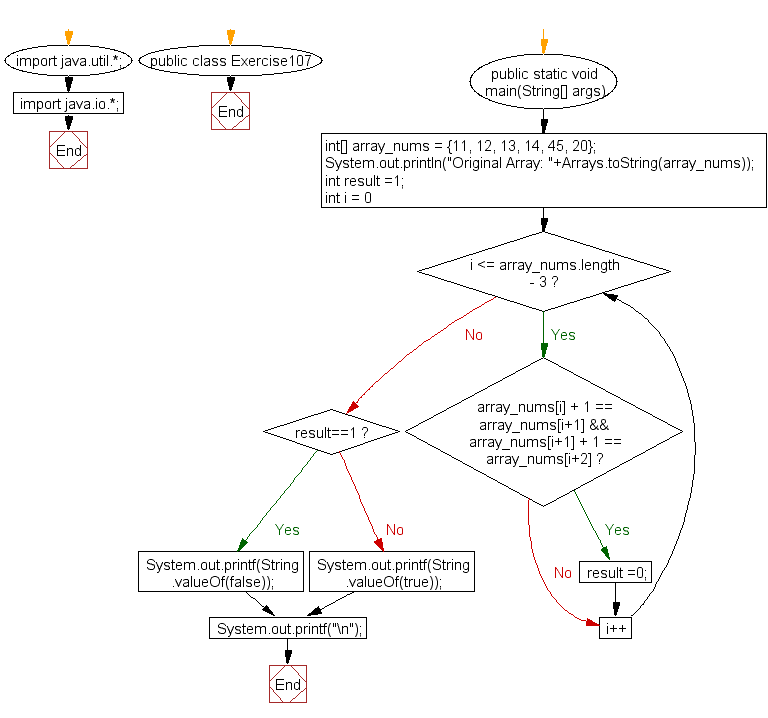﻿ Java: Check an array contains 3 increasing adjacent numbers# Java Exercises: Check if an array of integers contains three increasing adjacent numbers

## Java Basic: Exercise-107 with Solution

Write a Java program to check if an array of integers contains three increasing adjacent numbers.

Pictorial Presentation:Sample Solution:

Java Code:

``````import java.util.*;
import java.io.*;
public class Exercise107 {
public static void main(String[] args)
{
int[] array_nums = {11, 12, 13, 14, 45, 20};
System.out.println("Original Array: "+Arrays.toString(array_nums));
int result =1;

for(int i = 0; i <= array_nums.length - 3; i++) {
if(array_nums[i] + 1 == array_nums[i+1] && array_nums[i+1] + 1 == array_nums[i+2])
result =0;
}

if (result==1)
{
System.out.printf(String.valueOf(false));
}
else
{
System.out.printf(String.valueOf(true));
}
System.out.printf("\n");
}
}
```
```

Sample Output:

```Original Array: [11, 12, 13, 14, 45, 20]
true
```

Flowchart:Java Code Editor:

What is the difficulty level of this exercise?

Test your Programming skills with w3resource's quiz.

﻿

## Java: Tips of the Day

countOccurrences

Counts the occurrences of a value in an array.

Use Arrays.stream().filter().count() to count total number of values that equals the specified value.

```public static long countOccurrences(int[] numbers, int value) {
return Arrays.stream(numbers)
.filter(number -> number == value)
.count();
}
```

Ref: https://bit.ly/3kCAgLb